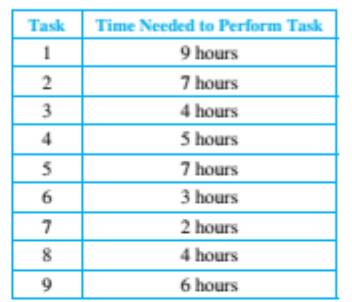Chapter 8.5, Problem 51ES### Discrete Mathematics With Applicat...

5th Edition
EPP + 1 other
ISBN: 9781337694193

#### Solutions

Chapter
Section### Discrete Mathematics With Applicat...

5th Edition
EPP + 1 other
ISBN: 9781337694193
Textbook Problem
1 views

# Suppose the tasks described in Example 8.5.12 require the following performance times:What is the minimum time required to assemble a car? Final a critical path for the assembly process.

To determine

(a)

To find out the minimum time required to assemble a car on the basis of the given table.

Explanation

Given information:

 Task Time needed to perform the task (in hours) 1 9 2 7 3 4 4 5 5 7 6 3 7 2 8 4 9 6

Calculation:

The following is the Hasse diagram drawn for the above table. In the diagram T1 means the Task 1 and 9h means 9 hours. The adjacent text represent the minimum number of hours to complete the tasks in the sequence

To determine

(b)

To find the critical path for the assembly process.

### Still sussing out bartleby?

Check out a sample textbook solution.

See a sample solution

#### The Solution to Your Study Problems

Bartleby provides explanations to thousands of textbook problems written by our experts, many with advanced degrees!

Get Started